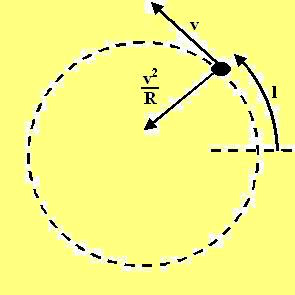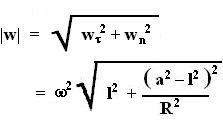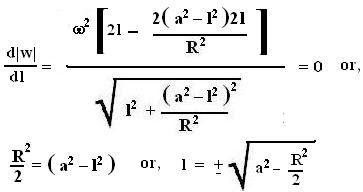## Sunday, July 22, 2007

### Irodov Problem 1.40

(a)
From the problem definition we can derive the tangential velocity and acceleration of the particle as,The centripetal acceleration that acts normal to the velocity isgiven by,The net acceleration is thus given by,Based on this expression it is easy to determine the accelerations at l=0 and l=a as,(b)
The value of l for which minimum value of acceleration occurs can be obtained by differentiating |w| with respect to l and equating to 0.The minimum acceleration can now be determined by substituting this obtained value of l as,